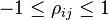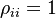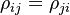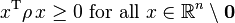# Correlation Matrix

## Definition

A Correlation Matrix denotes both a measure and a statistical of dependency (whether causal or not) between different stochastic processes

NB: This article focuses on aspects of correlation matrices relevant for risk management applications. More general statistical considerations are available in the wikipedia entry and links therein

### Alternative Correlation Measures

There are several alternative measures of correlation:

• The Pearson Correlation also referred to as Pearson's r, the Pearson product-moment correlation coefficient or the bivariate correlation, a measure of the linear correlation between two variables X and Y
• The Spearman's Rank Correlation a nonparametric measure of rank correlation (statistical dependence between the rankings of two variables)
• The Kendall Rank Correlation as Kendall's tau coefficient a statistic used to measure the ordinal association between two variables

## Properties

### Valid Range

All correlation matrix elements must satisfy$-1 \leq \rho_{ij} \leq 1$

The diagonal elements must be identical to unity$\rho_{ii} = 1$

### Symmetry

The matrix elements must satisfy the symmetry relation$\rho_{ij} = \rho_{ji}$

### Positive Semi-Definiteness

A correlation matrix must be positive semi-definite, namely$\quad x^{\mathrm T} \rho \, x \geq 0 \text{ for all } x \in \mathbb{R}^n \setminus \mathbf{0}$

When a matrix fails this requirement (usually due to Data Quality issues) one usually aims to find a nearby matrix that is valid. One approach to fix an invalid correlation matrix is provided here

An equivalent requirement is that all eigenvalues of the matrix are non-negative (See Definiteness of a matrix)

## Characterization

Correlation matrices are in general characterised according to the distribution of eigenvalues